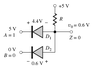# Diode AND Gate - Voltage Drop

• dobry_den

#### dobry_den

Hi! There's a schema of a diode AND gate attached. Could anyone of you, please, explain me why the voltage drop across the D1 diode is so big compared to the voltage drop across the D2 diode? Thanks a lot in advance!

#### Attachments

•AND Gate.GIF
2.5 KB · Views: 1,006

It says that the output voltage (=voltage at the black node) is 0.6V, but I can't really see how they came to this result...

Last edited:
One diode is in forward bias, and the other is in reverse bias. What limits the reverse bias voltage that you can put across a diode, BTW?

Oh I see, so the diode with 4.4V voltage drop is reverse biased then...? shame on me for not noticing that... And btw, when using the constant-voltage-drop model for a diode, does it also mean that the voltage drop across the resistor is also constant since it is connected with the diode in a series..? and to your question - i think i don't know what limits the reverse bias voltage across a diode.

Oh I see, so the diode with 4.4V voltage drop is reverse biased then...? shame on me for not noticing that... And btw, when using the constant-voltage-drop model for a diode, does it also mean that the voltage drop across the resistor is also constant since it is connected with the diode in a series..? and to your question - i think i don't know what limits the reverse bias voltage across a diode.

Yup, the 4.4V is reversed biased. In most cases the, the voltage drop across the resistor would be constant becuase of a constant current in the circuit. Temperature would have some effects, but usually very little.

Have you ever heard of breakdown voltage?

I meant the following..: assume A=1 (i.e. 5V) and B=0 (i.e. 0V). when the voltage drop across the diode is constant (say e.g. 0.6V), then - according to Kirchhoff's Voltage Law - the voltage drop across the resistor has to be constant as well (4.4V in this case), independently of its resistance. The current will change with the change of resistance (I = U/R), but the voltage drop (U) will remain the same*. Is this reasoning correct?

* The resistance will of course have to remain in a certain interval, since the constant-voltage-drop model of a diode is restricted to a certain interval of current magnitudes (like 1mA to 10mA).

Last edited:
Hope I understand the notion of breakdown voltage correctly - it is the minimal voltage drop across a reverse biased diode that makes the diode conduct.

And one more question - if both inputs are 5V, then D1 and D2 diodes are reverse biased and the output is equal to (5V - voltage_drop_across_the_resistor) instead of 5V, isn't it?

So when creating these diode gates, should I use resistors with low resistance in case of an AND gate and high resistance in case of an OR gate?